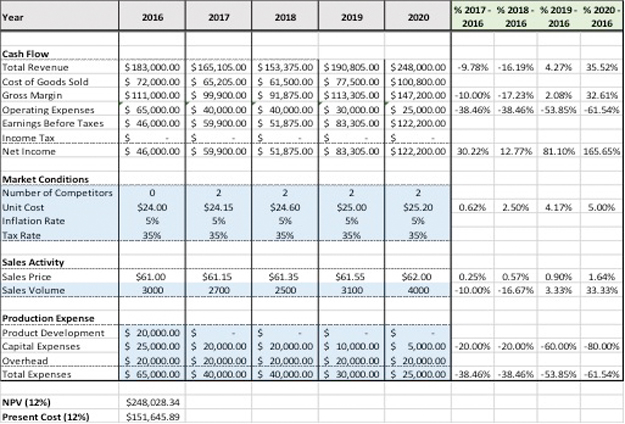# What is Financial Modeling?

Definition: Financial modeling refers to the building of mockups with variables and calculations that aim to emulate and explain a firm’s or a portfolio’s performance.

## What Does Financial Modeling Mean?

Financial modeling translates a set of assumptions about a particular business event to a set of numerical projections. The model captures all the variables, and quantifies them to produce a mathematical interpretation of the business event on Excel spreadsheets.

In that way, financial analysts comprehend the event and can accurately estimate a firm’s future growth. Often, a financial model summarizes the findings, suggesting a required, or an alternative action. The most commonly used financial models are the capital asset pricing model (CAPM), the dividend discount model (DDM), the discounted cash flow model (DCF), and more sophisticated models such as the leveraged buyout model (LBO) and the merger & acquisition model (M&A).

Let’s look at an example.

## Example

Gus is a financial analyst at a manufacturing company. The company has recently undertaken a new project, and Gus is asked to calculate the projected growth earnings. Gus is very experienced with Excel, so he creates a financial model. This is how the model looks like:Gus calculates the net present value of the project compared to the cost of the project using the NPV function in Excel. He finds that the project is rightfully undertaken as the NPV is \$248 million and the cost of the project is \$151.65. How has Gus concluded this?

He calculates the projected revenues from 2017 to 2020 assuming different sales volumes multiplied by different sales prices. So, for 2016 he estimates 3,000 x \$61 = \$183,000, for 2017 2,700 x \$61.15 = \$165,105, and so on.

Then, he calculates the cost of goods sold through 2020 by multiplying the unit cost x sales volume. The inputs in the blue cells are based on realistic scenarios, on figures that the company can achieve.

Then, he calculates the operating expenses, which are the total expenses calculated at the end of the model through 2020, and represent the total cost of the project.

Finally, he finds the net income through 2020, which is used to calculate the NPV of the project.

After the projections, Gus calculates the projected growth in revenues, gross margin, operating expenses, and net income. He does the same for the unit cost, sales price, and sales volume as well as for the expenses. Now, he can present his conclusions to his manager.